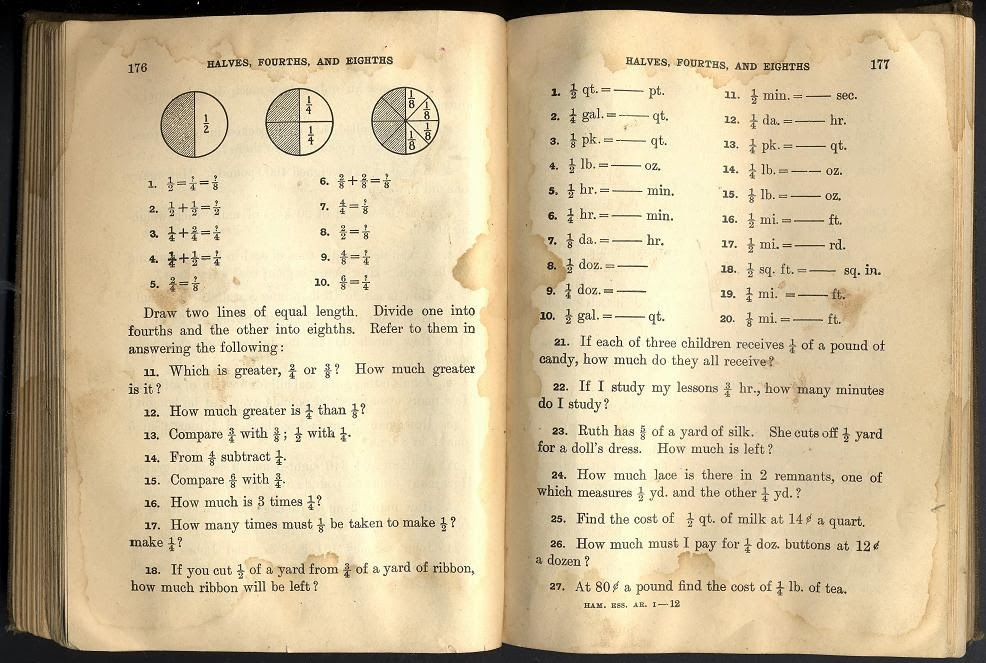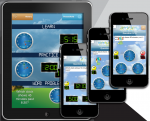@Reks: Educational iOS Applications

## Tick Tock Clock: Learn to Tell TimeTick Tock Clock: Learn to Tell Time – FREE is the latest educational and entertaining iOS application designed and created by AtReks. The app is made FREE for all the teachers, educators, parents and students who would like to see a sample app first before deciding to buy a full version of the same app. Full version […]

## 2nd Grade Printable Math Quiz Workshe...You can download a number of math practice worksheets designed for Grade 2  Elementary School. The practice worksheets are based on Math Essentials textbook and math common core standards. Unit 1 covers the following concepts: add and subtract numbers up to 20 add three or four numbers find partners and totals write equations for math mountains tell […]

## 5th Grade Printable Math Quiz SheetsThis page offers a number of math worksheets for Grade 5 based on Math Essentials math textbook and common core standards. Unit 1 covers the following topics: – fractions – simplify fractions – addition and subtraction of fractions – word problems with fractions Tap/click the following links to print math tests from Unit 1: 1. 5th Grade Unit 1 […]This page offers a number of math worksheets for Grade 4 following Math Essentials math textbook and common core standards. The worksheets are organized in four different lessons (Units). The Math topics in the first lesson (Unit) are as follows: – Calculate area and perimeter of triangle, square, rectangle, parallelogram, or trapezoid – Order of […]

## Learn to Tell Time With Ultimate Edu ...The latest update of Tick Tock Clock – Learn How to Tell Time Using Digital and Analog Clock with Roman and Arabic Numerals in iTunes as of recently.  What’s New in Version 3.0: ► Now 3 MODES: LEARN, PRACTICE and WORD PROBLEMS ► WORD PROBLEMS test mode includes an option to choose level of difficulty […]